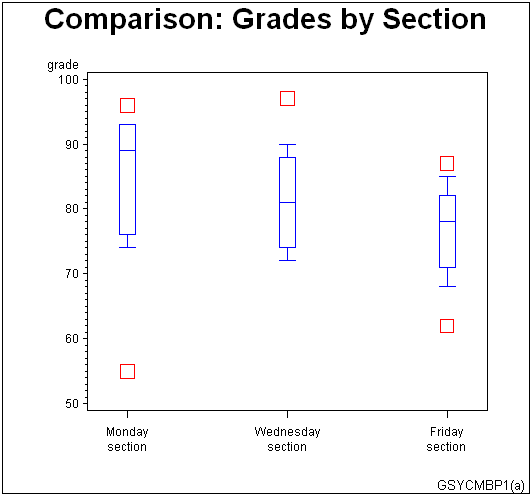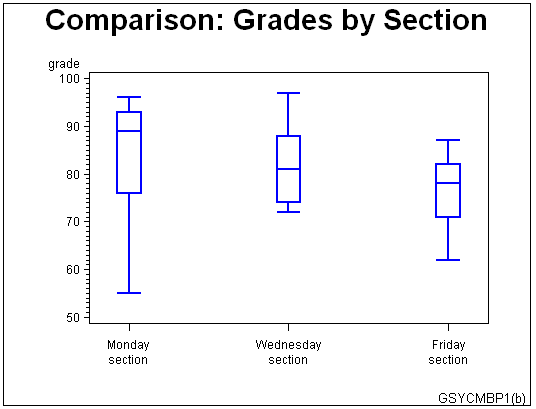SAS/GRAPH Statements

# Example 4. Creating and Modifying Box Plots

Features:

SYMBOL statement options:

 BWIDTH= CO= CV= HEIGHT= INTERPOL= VALUE=

Sample library member: GSYCMBP1This example shows how to create box plots and how to specify SYMBOL definitions so data outside the box-plot range can be represented with data points. It also shows how to change a box plot's percentile range to see whether the new range encompasses the data.

The first plot in the example uses a SYMBOL definition with INTERPOL=BOXT20 to specify a box plot with whisker tops at the 80th percentile and whisker bottoms at the 20th percentile. Data points that are outside this percentile range are represented with squares.

As illustrated in the following output, the example then changes the SYMBOL definition to INTERPOL=BOXT10, which expands the whisker range to the 90th percentile for tops and the 10th percentile for bottoms. There are no data points outside the new percentile range.Set the graphics environment.

`goptions reset=all border;`

Create the data set. GRADES contains codes to identify each class section, and the grades scored by students in each section.

```data grades;
datalines;
A 74 A 89 A 91 A 76 A 87 A 93 A 93 A 96 A 55
B 72 B 72 B 84 B 81 B 97 B 78 B 88 B 90 B 74
C 62 C 74 C 71 C 87 C 68 C 78 C 80 C 85 C 82
;```

Define title and footnote.

```title1 "Comparison: Grades by Section";
footnote1 j=r  "GSYCMBP1(a) ";```

Define symbol characteristics. INTERPOL=BOXT20 specifies a box plot with tops and bottoms on its whiskers, and the high and low bounds at the 80th and 20th percentiles. The CO= option colors the boxes and whiskers. The BWIDTH= option affects the width of the boxes. The VALUE= option specifies the plot symbol that marks the data points outside the range of the box plot. The CV= option colors the plot symbols. The HEIGHT= option specifies a symbol size.

```symbol interpol=boxt20 /* box plot              */
co=blue         /* box and whisker color */
bwidth=4        /* box width             */
value=square    /* plot symbol           */
cv=red          /* plot symbol color     */
height=2;       /* symbol height         */```

Define axis characteristics.

```axis1 label=none
value=(t=1 "Monday" j=c "section"
t=2 "Wednesday" j=c "section"
t=3 "Friday" j=c "section")
offset=(5,5)
length=50;```

Generate the first plot.

```proc gplot data= grades;
vaxis=50 to 100 by 10;
run;```

Define the footnote for the second plot.

`footnote j=r "GSYCMBP1(b)";`

Change symbol characteristics. INTERPOL=BOXT10 changes the high and low bounds to the 90th percentile at the top and the 10th percentile on the bottom. All other symbol characteristics remain unchanged.

`symbol interpol=boxt10 width=2;`

Generate the second plot.

```plot grade*section / haxis=axis1
vaxis=50 to 100 by 10;
run;
quit;```Previous Page | Next Page | Top of Page